# Effect of Combined Circuit

This page shows the elaboration of the issue on the page: series and parallel.

Because I assume that people understand the theory and have practiced it themselves, I only give a brief explanation and answers about the following circuit on this page: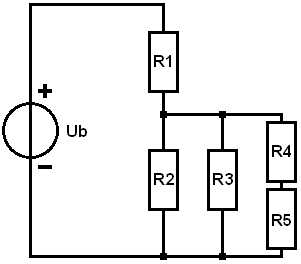1. Determine the RV of the parallel connection:
The parallel circuit contains a series circuit, so R4 and R5 are added together for convenience. Actually, we could consider this as the third Rv in the circuit. Because it then becomes confusing with the accents, we leave this out.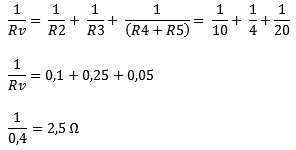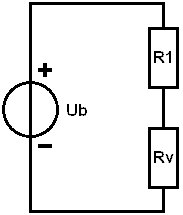2. Determine Rv of the series connection:
The total replacement resistance of the combined series and parallel circuit is called Rv'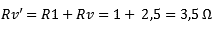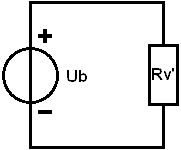3. Calculate Itotal:4. Calculating partial voltages: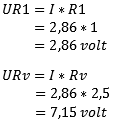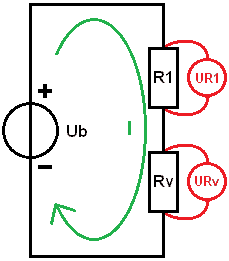5. Calculating Flows: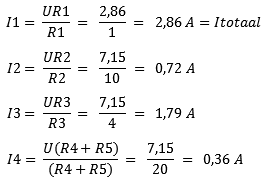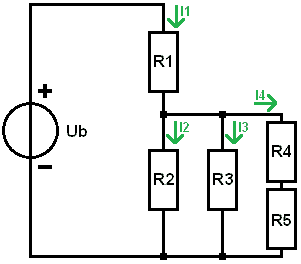6. Calculate partial voltages UR4 and UR5 + the total overview.An addition of these partial voltages must be equal to the voltage across R3.

The image shows the complete overview with currents and voltages. To practice this yourself, you can change one resistance value and go through all the steps again.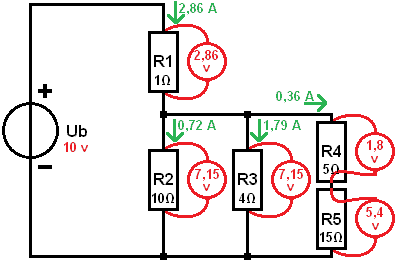error: Alert: Content is protected !!